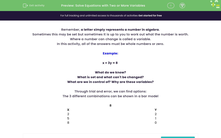# Solve Equations with Two or More Variables

In this worksheet, students will be asked to solve equations with two or more variables.Key stage:  KS 2

Curriculum topic:   Algebra

Curriculum subtopic:   Use Variables

Popular topics:   Algebra worksheets

Difficulty level:#### Worksheet Overview

Remember, a letter simply represents a number in algebra.

Sometimes this may be set but sometimes it is up to you to work out what the number is worth.

Where a number can change it is called a variable.

In this activity, all of the answers must be whole numbers or zero.

Example:

x + 3y = 8

What do we know? That the total must be 8.

What is set and what can't be changed? The answer is set and that y needs to be multiplied by 3.

What are we in control of? Why are these variables? x and y are the variables because they could be several different numbers.

Through trial and error, we can find options:

The three different combinations can be shown in a bar model

 8
 X Y 2 2 5 1 8 0

Option 1: If x is 2, then 3y must be 6 so that they add up to 8. If 3y is 6, then y is 2.

Option 2: If x is 5, then 3y must be 3 so that they add up to 8. If 3y is 3, then y is 1.

Option 3: If x is 8, then y must be 0 so that they add up to 8.

Let's have a look at the questions now.

### What is EdPlace?

We're your National Curriculum aligned online education content provider helping each child succeed in English, maths and science from year 1 to GCSE. With an EdPlace account you’ll be able to track and measure progress, helping each child achieve their best. We build confidence and attainment by personalising each child’s learning at a level that suits them.

Get started